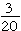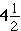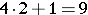# Multiplication of Fractions and Mixed Numbers

In this lesson, you will review fraction and mixed number multiplication as you learn to apply this skill to real life situations involving both positive and negative rational numbers. Have you ever mixed ingredients for a recipe? Fractions are a normal part of the ingredients. What if you had to double the recipe, or cut it in half? Multiplying fractions is necessary for this type of purpose.

To multiply fractions, it is simple. Just multiply the numerators, multiply the denominators, then simplify!

Example:Multiply 3 * 1 and Multiply 5 * 4 =You can also look for common factors and simplify BEFORE you multiply! Check out the example below to see how this works!Before you can multiply mixed numbers, you must convert them into improper fractions. One common method is called the Texas Method, which just stands for tx (t is like a plus sign, x is like a multiplication sign), meaning you have two operations required – you must use addition and multiplication. If you were to convertto an improper fraction, you would work clockwise and multiply the denominator by the whole number, than add that total to the numerator. Therefore,. The denominator does not change, so you have. Then you should simplify your fractions.

REMEMBER to turn a whole number into a fraction with a denominator of 1 before multiplying. A common error is to multiply the whole number by the mixed number and just keep the fraction. You must always turn mixed numbers into improper fractions when multiplying it with any number.

(source)